## Flyback converter

##### Introduction

The objective of this experiment is to study the characteristics of a flyback converter. The circuit will be operated under both continuous conduction mode (CCM) and discontinuous conduction mode (DCM).

##### Theoretical background

The flyback converter is derived from buck-boost converter shown below.The inductor in the buck-boost converter, shown in the above image with its low permeability core, is replaced with two mutually coupled coils as shown.The above circuit is typically drawn as shown below with the two mutually coupled coils represented using a transformer.Turning on the transistor increases the primary current, thereby the energy stored in the mutually coupled coils. During this period, the voltage across the transformer primary Vpri = Vin, and the secondary side voltage Vsec = -(N2 / N1) × Vin. The voltage across the diode VD = Vo - Vsec = Vo + (N2 / N1) × Vin, which reverse biases the diode.

The transformer coils are oriented such that when the transistor is turned off the current switches from the primary to the secondary coil to maintain the flux in the core. This current forward biases the diode, thus during the transistor off period the voltage across the transformer secondary Vsec = Vo. During this period, the voltage across the primary Vpri = -(N1 / N2) × Vo and the voltage across the transistor Vsw = Vin + (N1 / N2) × Vo.

For a given transistor switching function waveform q(t) with a switch duty ratio d in steady state, the waveform of the converter voltages and currents are as shown.When the switch is ON, i.e., q(t) = 1, the change in the transformer flux Δφm is given by,

Δφm = VinN1 × d × Ts(1)

When the switch is OFF, i.e., q(t) = 0, the change in the transformer flux Δφm is given by,

Δφm = VoN2 × (1 - d) × Ts(2)

At steady-state under CCM, the change in flux during the turn ON and OFF period must be equal. Thus equating Eqns. 1 and 2

Vo = N2N1 × d1 - d × Vin(3)

Flyback converter operation under DCM is quite similar to that of buck-boost converter under DCM. In case of buck-boost converter the boundary condition between CCM and DCM was when the inductor current briefly goes to zero. In case of flyback converter the boundary condition is when the flux in the core briefly goes to zero. For a given transistor switching function waveform q(t), shown below, with a switch duty ratio d in steady state under DCM, the waveform of the voltage across the transitor Vsw, for an ideal flyback converter, is as shown below.When the switch is ON, i.e., q(t) = 1, Vsw = 0. When the switch is OFF but the flux in the transformer core φm or the current in the secondary winding current isec is not yet zero, Vsw = Vin + (N1 / N2) × Vo. This is because the diode is conducting during this period, hence the output voltage gets impressed across the transformer secondary winding. When the switch is OFF and the flux in the transformer core φm is zero, Vsw = Vin. This is because the diode is no longer conducting and it blocks the output across it.

Many flyback converters typically operate under DCM. This is because once the flux in the core goes to zero, the voltage across the switch is lower than when the flux was non-zero. Thus turning on the switch under DCM leads to lower switching losses compared to CCM. In a practical flyback converters this effect is more pronounced due to the ringing in the voltage across the transistor, due to parasitic components as discussed in Buck-boost converter - Discontinuous conduction mode (DCM), as shown below.When the flux goes to zero, the voltage across the transistor, ignoring damping, can go low as Vv = Vin - (N1 / N2) × Vo, clamped at 0 V. Practical flyback converters are typically designed to turn ON the transistor at this valley to signifcantly reduce the switching losses.

##### Preparing the Workbench model
1. Copy the folder where pre-built example project for this experiment is present, usually in C:\Program Files (x86)\Sciamble\WorkBench v1\Examples\CUSPLab\BasicPowerElectronics\Experiment11 and paste it in a location where the user has permission to edit and save files, like the Desktop folder.

2. Launch Workbench application.

3. Pin (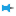) the Explorer dock on the right and the Toolbox dock on the left.

4. Click the second icon within the Explorer dock,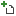to open the project.

5. Navigate to the folder where the pre-built example project was pasted in Step 1. Double click the FlybackConverter.project node within that folder to open the project.

6. Click the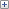icon to explore the files within the project. Double click the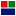Flyback node in the Explorer dock to display the model file.

7. Ensure that the switching frequency is set to 100 kHz. To do this, double click on the project node,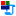FlybackConverter, in the Explorer. Go to the next page by clicking the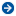in the property dock or choosing Device configuration from the drop-down menu. Check that the Frequency within PWM Configuration is set to 100000.

8. Ensure that the PWM channel is set to the Si power pole. To do this, open the property of the tool labelled Si PWM in the model by double clicking on it. Check that the Channel is set to 1.

##### Preparing the setup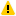Warning

Before proceeding, ensure that the isolated power supply is powered down and that the USB cable is disconnected.

1. Magnetics card connection: Unscrew any existing magnetics card and replace it with the Flyback magnetics card. Make sure that all the 6-pins of the magnetics card are making solid contact with the power-pole board.

Prior to connecting the card, ensure that the jumper on the bottom of the magnetics card is connected. This is to connect the snubber circuit across the transformer primary to clamp the ringing across the transistor. The theory of operation and the procedure to design the snubber will be dealt in the next experiment, Flyback converter snubber design.Warning

Never leave the magnetics card unscrewed. If contact is lost while the converter is running, it has the potential to cause very high voltage due to interruption of inductor current, potentially damaging the converter, or worse, could lead to a safety hazard.

2. Rheostat setting: Set the slider such that the resistance across the two closest rheostat terminals is 8 Ω.

3. Power connections:

1. Connect the Vin terminals to the isolated power supply:

1. DC +ve: Vin+ (Red)

2. Ground: Earth (Green)

3. `DC −ve: ● Vin− (Black)`

2. Connect the Vo terminals to the rheostat load:

1. Rheostat rail: Vo+ (Red)

2. `Rheostat's other terminal that is closest to the rail: ● Vo− (Black)`

4. DSO connections:

1. Connect DSO channel 1 probe to Io. Set the following options, if they are supported by the DSO:

1. Set the probe to 1x.

2. Set the measurement type as Current.

3. Set the scaling factor to 5x.

4. Set the offset at 1.5 V or 7.5 A.

5. Set termination to 1 MHz.

6. Set the channel as inverted.

2. Connect DSO channel 2 probe to SWA. Set the following options, if they are supported by the DSO:

1. Set the probe to 1x.

2. Set the measurement type as Voltage.

3. Set the scaling factor to 1x.

4. Set the offset at 0 V.

5. Set termination to 1 MHz.

6. Set the channel as default/non-inverted.

3. Connect DSO channel 3 probe to Iin. Use the same setting as that of Channel 1.

4. Connect DSO channel 4 probe to Vout. Use the same setting as that of Channel 2.

5. Jumper settings:

1. Disconnect the Vdc jumper.

2. Insert the GaN/Si jumper to the Si side.

3. Insert the GaN switch's external diode jumper, labelled "Diode".

4. Insert the jumper on the bottom of the magnetics card.

6. Connect the USB cable to the power-pole board and the computer.

7. DC power supply settings:

1. Make sure that the DC power supply is fully turned down to 0 V prior to turning on the supply.

2. Turn on the power supply and gradually ramp up the voltage from 0 V to 15 V.

3. If the option is available, set the power supply current limit at 4.5 A.

The final wiring should look similar to this: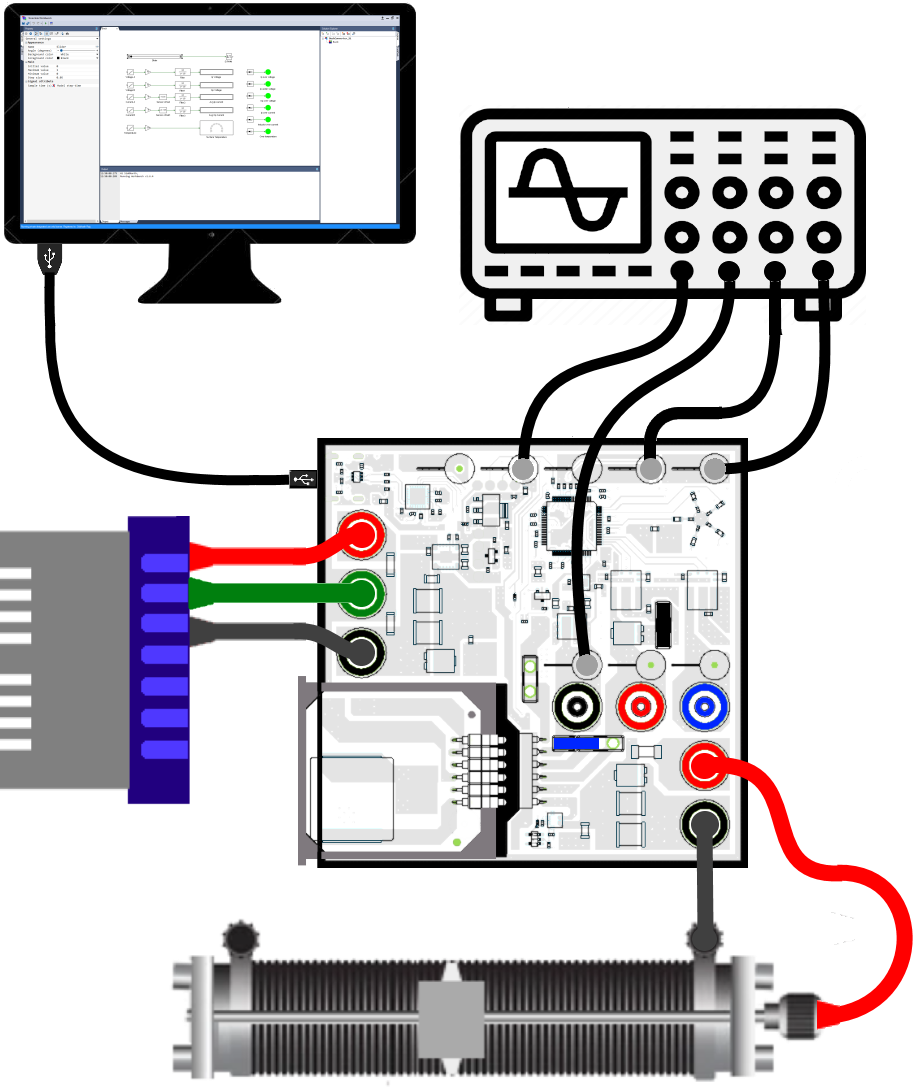Warning

The input and output of a typical flyback converter is galvanically isolated. That is not the case in this lab kit.To enable both external measurement as well as measurment by the controller of the input, the output, and the switch voltages without the need for measurement isolation, the output negative is internally connected to the input negative. Thus the output is not electrically isolated. This does not alter the response of the converter in any manner.

##### Real-time open loop control of flyback converter - with snubber
Running the setup:
1. Click on the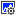icon in the top dock of Workbench to transition from the simulation mode to the real-time mode.

2. Click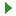to run the control algorithm in real-time.

3. If an undervoltage fault occurs, slightly increase the input voltage above 15 V but less than 16 V. Stop the model by clicking on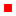and rerun it.

4. Compensate the offset in the current sensors. To do this, make note of the value, up to 3 decimal places, displayed on the Avg Ip Current and the Avg Op Current tool.

5. Click onto stop the model.

6. Open Sensor offset tool's property by double clicking on it and set the Offset value to the negative of the measured Avg Ip Current in the previous step.

Open Sensor offset1 tool's property and set the Offset value to the negative of the measured Avg Op Current in the previous step.

7. Clickto rerun model.

8. Once programmed, ensure that the Avg Ip Current and the Avg Op Current both initally display a value of 0 for up to 2 decimal places. If not, repeat the previous 3 steps.

9. Gradually increment the duty cycle from 0 to 0.5 in steps of 0.05. If a fault occurs, stop the model by clicking onbutton.

10. Make the following measurements:

1. Observe the DSO waveforms and make a copy of the voltage across the switch (channel 2), the output voltage (channel 4), the input/primary current (channel 1), and the diode/secondary current (channel 3) for duty cycle of 0.1, 0.2, 0.3, 0.4 and 0.5 (or the highest possible value prior to when the fault occured). Adjust the time base to show anywhere between 4-10 switching cycles.Warning

Do not exceed duty cycle of 0.5. Even though the input and output voltages, which are monitored by the controller, will be within safe limits, the switch voltage, which is not monitor by the controller, could exceed the device rating for duty greater than 0.5. This is because the voltage across the switch is the sum of input voltage and the reflected output voltage.

2. Make a note of the voltage and current values displayed on Workbench, for each duty cycle step.

11. Click onto stop the model.

If required, repeat the same experiment using GaN power-pole instead of Si power-pole as demostrated in Switching characteristic of Si MOSFET/GaN FET and diode.

##### Lab report and reading assignment
1. Attach the DSO waveforms showing the voltage across the transistor, the output voltage, the inductor current, and the input current for switching frequency of 100 kHz and duty cycles of 0.1, 0.2, 0.3, 0.4, and 0.5.

2. From these waveforms calculate the tranformer turns ratio N1 : N2.

3. For switching frequency of 100 kHz and duty cycle varying from 0.1 to 0.5 in steps of 0.05, enter the measured values from the Workbench screen capture and calculate the following values:

dset Vin (V) Iin,avg (A) Pin (W) Vo (V) Io,avg (A) Pout (W) dact Efficiency (%)
`        `
`        `
`        `
`        `
`        `
`        `
`        `
`        `
`        `

where, dset is the duty cycle value set using Workbench and dact is the duty cycle (d) calculated using Eqn. 3.

Plot the efficieny as a function of dutyact.

Plot the Vo as a function of dutyset.

4. From the plot of primary current at 100 kHz switching frequency, duty cycle of 0.5 (or the maximum duty prior to fault), estimate the transformer magnetizing inductance.

##### Reference
1. "Power Electronics, A First Course," Ned Mohan and Siddharth Raju, Wiley Publication.

< Voltage mode control : previous topic